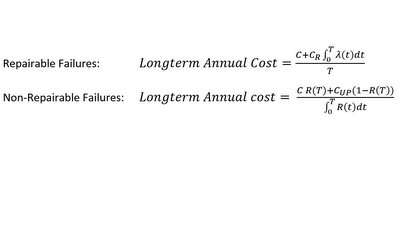# Fundamental Equations

Alec Erskine explores how some key equations can assist with the business of asset management in waterMost people say that you should never put equations in a report, presentation or blog. I do not subscribe to this view. In fact I think that equations are good for you. So there’s a couple of crackers at the top of this blog.

Many areas of science have fundamental equations. The greatest physicists the world has ever seen have left their indelible stamp on our understanding of the universe through equations: Newton’s gravity equation, Einstein’s E=mc2, and James Clerk Maxwell’s four electro-magnetism equations (explaining simultaneously how light works and how magnetic fields create electricity). Most subjects have an equation or two that is central to their philosophy and calculation - perhaps Colebrook-White is the one most water engineers hold dear. So what about asset management?

Asset management is about making decisions about managing assets. We try to do this quantitatively using asset behaviour models and cost knowledge and our economic framework to time our interventions optimally. With this in mind, I propose that the two equations below should be the fundamental equations of asset management. In both, λ(t) is the failure rate at age t and embodies the asset deterioration model. The first equation is the long-term spend rate with replacement age T for repairable assets and the second is the long-term spend rate for non-repairable assets (i.e. “fatal” failures). Change T until you get the minimum value and you’ve got the optimal replacement time. In reliability engineering, this is known as the age replacement problem. Many asset management decisions can be made on the basis of some version of these equations.

The equations are fairly simple to derive. Repairable failures (e.g. sewer blockages) get fixed as they happen and the assets last as long as you like (in this model anyway). In every cycle of time T, you pay the capital cost C and have a total risk of consequence CR (repair cost) times total likelihood from age 0 to T.

The second equation is about non-repairable failures that end the asset’s life, so now we have the reliability R(t), defined as the likelihood of the asset being still working at age t. There’s a chance (1-R(T) in fact) that the asset fails before reaching T. The driver now is the contrast between how much you pay for a replacement, C, versus how much you pay for an unplanned replacement when it fails, CUP (including all the extra consequences). Note that if CUP is the same as C, then you minimise C/∫R(t)dt which means maximising ∫R(t)dt which means T is infinity – this is a “run-to-fail” policy.

In civil engineering, we often have to think many years ahead and the cost of money becomes important. Both of the equations above do have versions involving discounting, which effectively push the replacement times further out. They are a bit frightening so I haven’t included them in the blog, but it could be argued that public-service organisations should be catering for the very long-term and therefore shouldn’t need discounting. It could also be argued that they have to borrow money, the same as anybody else, and therefore should be including discounting.

These equations contrast with the engineer’s traditional Cost-Benefit calculation which tends to go along the lines of: Replace if Cost < NPV(Probability of failure × Consequence of failure) with NPV being calculated with a fixed horizon of 30 years. Maybe next blog I’ll investigate how far wrong this approach can go.

And since you ask, I do get out sometimes.